GeeksforGeeks App
Open AppBrowser
Continue

# Class 10 RD Sharma Solutions – Chapter 3 Pair of Linear Equations in Two Variables – Exercise 3.2 | Set 1

### 2x + 5y = 12

Solution:

Given that, 2x + 5y = 12 and x + y = 3

We have,

x + y = 3,

When y = 0, we get x = 3

When x = 0, we get y = 3

So, the following table giving points on the line x + y = 3:

Now, 2 + 5y = 12

y = (12 – 2x)/5

When x = 1, we have

y = (12 – 2)/5 = 4

When x = -4, we have

y = (12 – 2(-4))/5 = 4

So, the following table giving points on the line 2x + 5y = 12:

So, the graph of the equation x + y = 3 and 2x + 5y = 12 isFrom the graph we conclude that the two lines intersect at a point P (1, 2).

Hence x = 1 and y = 2 are required point.

### 2x + 3y = 10

Solution :

Given that,, x – 2y = 5 and 2x + 3y = 10

Now, x – 2y = 5

x = 5 + 2y

When y = 0, we get x = 5

When y = -2, we get x = 1

So, the following table giving points on the line x – 2y = 5

Now,  2x + 3y = 10

x = (10 – 3y)/2

When y = 0, we get x = 5

When y = 2, we get x = 2

So, the following table giving points on the line 2x + 3y = 10

So, the graph of the equation x – 2y = 5 and 2x + 3y = 10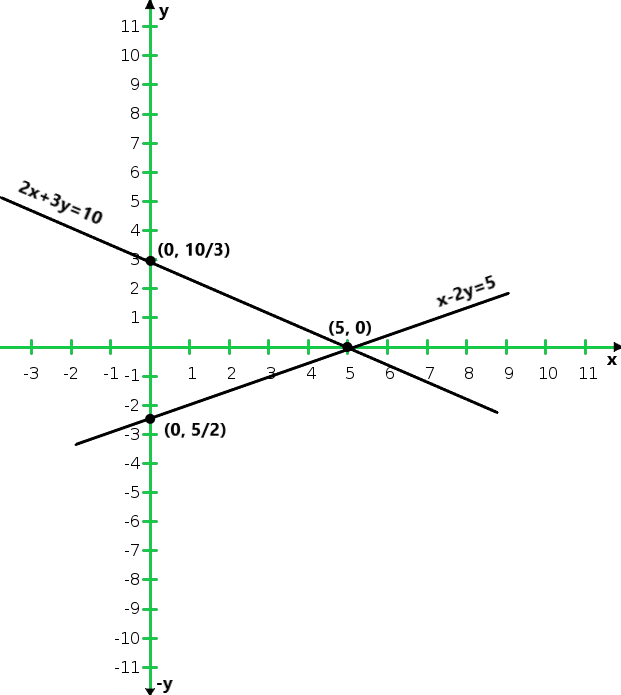From the graph we conclude that the two lines intersect at a point P (5, 0)

Hence x = 5 and y = 0 are required point.

### 2x – 3y + 8 = 0

Solution:

Given that, 3x + y + 1 = 0 and 2x – 3y + 8 = 0

Now 3x + y + 1 = 0

y = -1 – 3x

When x = 0, we get x = -1

When y = -1, we get x = 2

So, the following table giving points on the line x – 2y = 5

Now,  2x – 3y + 8 = 0

x = (3y – 8)/2

When y = 0, we get x = -4

When y = 2, we get x = 1

So, the following table giving points on the line 2x + 3y = 10

So, the graph of the equation 3x + y + 1 = 0 and 2x – 3y + 8 = 0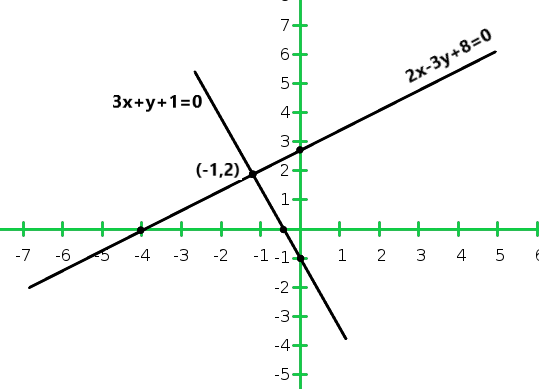From the graph we conclude that the two lines intersect at a point P (-1, 2)

Hence x = -1 and y = 2 are required points.

### 2x – 3y – 7 = 0

Solution:

Given that,, 2x + y – 3 = 0 and 2x – 3y – 7 = 0

Now 2x + y – 3 = 0,

y = 3 – 2x

When x = 0, we get x = 3

When x = 1, we get x = 1

So, the following table giving points on the line 2x + y – 3 = 0

Now,   2x – 3y – 7 = 0

When x = 0, we get y = 1

When x = 2, we get y = -1

So, the following table giving points on the line 2x + 3y = 10

So, the graph of the equation 2x + y – 3 = 0 and 2x – 3y – 7 = 0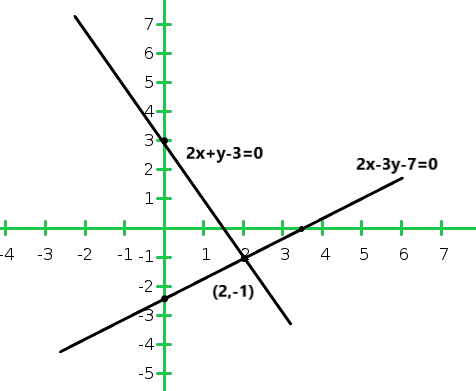From the graph we conclude that the two lines intersect at a point P (2,-1)

Hence x = 2 and y = -1 are required points.

### x – y = 2

Solution:

Given that, x + y = 6 and x – y = 2

Now x + y = 6

y = 6 – x

When x = 2, we get y = 4

When x = 3, we get y = 3

So, the following table giving points on the line x + y = 6

Now ,  x – y = 2

y = x – 2

When x = 0, we get y = – 2

When x = 2, we get y = 0

So, the following table giving points on the line x – y = 2

So, the graph of the equation x + y = 6 and x – y = 2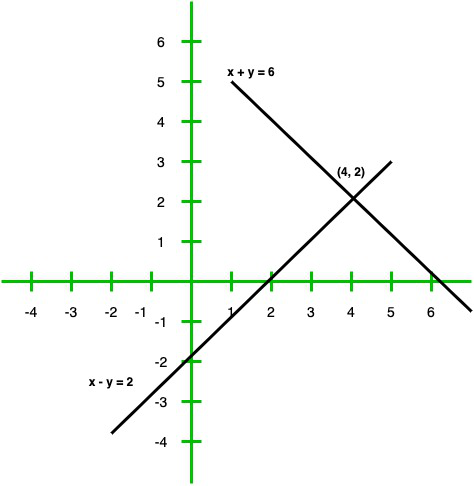From the graph we conclude that the two lines intersect at a point P (4, 2)

Hence x = 4 and y = 2 are required points.

### 3x – 6y = 0

Solution:

Given that,   x – 2y = 6 and 3x – 6y = 0

Now x – 2y = 6

x = 6 + 2y

When y = -2, we get x = 2

When y = -3, we get x = 0

So, the following table giving points on the line x – 2y = 6

Now,  3x – 6y = 0

= x = 2y

When y = 0, we get y = 0

When y = -1, we get x = 2

So, the following table giving points on the line 3x – 6y = 0

So, the graph of the equation x – 2y = 6 and 3x – 6y = 0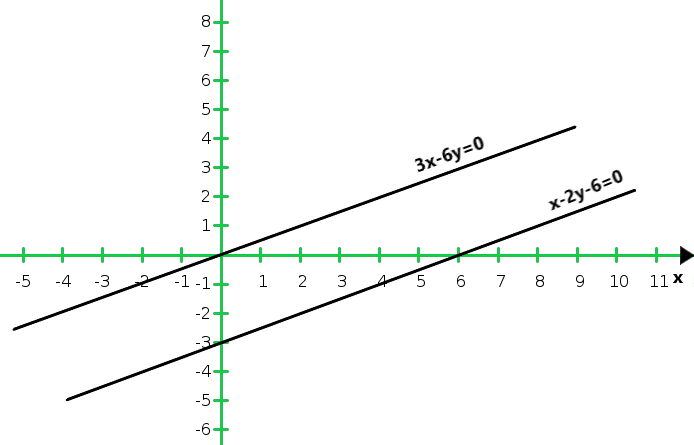From the graph we conclude that the two lines are parallel to each other so, the two lines have no common point.

Hence the given system has no solutions.

### 2x – 3y = 3

Solution:

Given that,   x + y = 4 and 2x – 3y = 3

Now x + y = 4

x = 4 – y

When y = 0, we get x = 4

When y = 2, we get x = 2

So, the following table giving points on the line x + y = 4

Now ,  2x – 3y = 3

x = (3y + 3)/2

When y = 1, we get x = 3

When y = -1, we get x = 0

So, the following table giving points on the line 2x – 3y = 3

So, the graph of the equation x + y = 4 and 2x – 3y = 3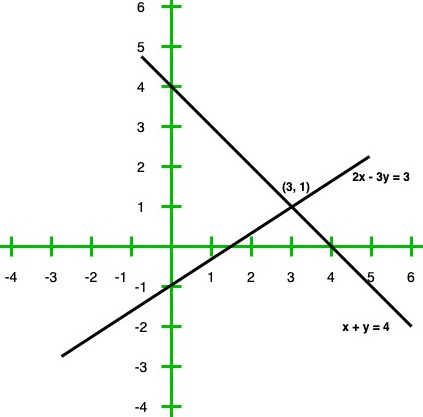From the graph we conclude that the two lines intersect at a point P(3, 1)

Hence x = 3 and y = 1 are required points.

### x – y + 3 = 0

Solution:

Given that, 2x + 3y = 4 and x – y + 3 = 0

Now 2x + 3y = 4

x = (4 – 3y)/2

When y = 0, we get x = 2

When y = 2, we get x = – 1

So, the following table giving points on the line x + y = 4

Now,  x – y + 3 = 0

x = y – 3

When y = 3, we get x = 0

When y = 4, we get x = 1

So, the following table giving points on the line x – y + 3 = 0

So, the graph of the equation 2x + 3y = 4 and x – y + 3 = 0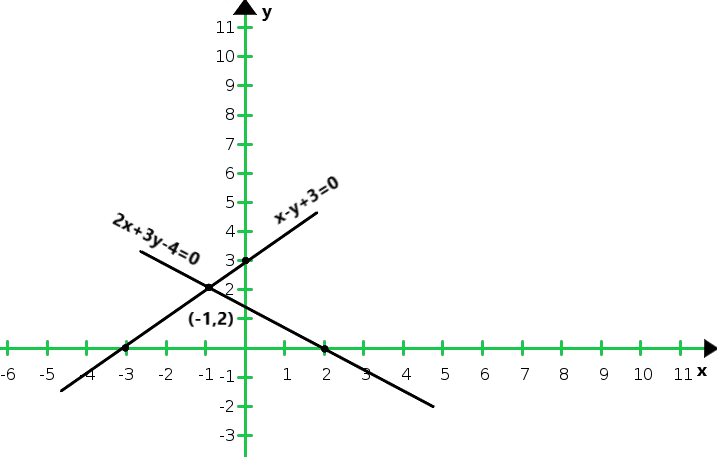From the graph we conclude that the two lines intersect at (-1, 2)

Hence x = -1 and y = 2 are required points.

### 3x – 2y + 12 = 0

Solution:

Given that, 2x – 3y + 13 = 0 and 3x – 2y + 12 = 0

Now,  2x – 3y + 13 = 0

x = (3y – 13)/2

When y = 1, we get x = – 5

When y = 3, we get x = – 2

So, the following table giving points on the line 2x – 3y + 13 = 0

Now,  3x – 2y + 12 = 0

x = (2y – 12)/3

When y = 0, we get x = -14

When y = 3, we get x = -2

So, the following table giving points on the line x – y + 3 = 0

So, the graph of the equation 2x – 3y + 14 = 0 and 3x – 2y + 12 = 0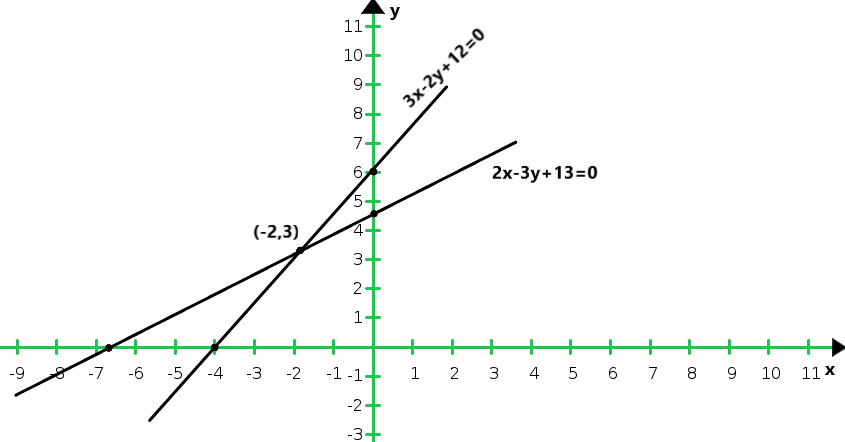From the graph we conclude that the two lines intersect at (- 2, 3)

Hence x = – 2 and y = 3 are required points.

### 3x – 2y – 12 = 0

Solution:

Given that, 2x + 3y + 5 = 0 and 3x – 2y – 12 = 0

Now,  2x + 3y + 5 = 0,

x = (-3y – 5)/2

When y = 1, we get x = – 4

When y = -1, we get x = -1

So, the following table giving points on the line 2x + 3y + 5 = 0

Now,  3x – 2y – 12 = 0,

x = (2y + 12)/3

When y = 0, we get x = 4

When y = 3, we get x = 6

So, the following table giving points on the line 3x – 2y – 12 = 0

So, the graph of the equation 2x + 3y + 5 = 0 and 3x – 2y – 12 = 0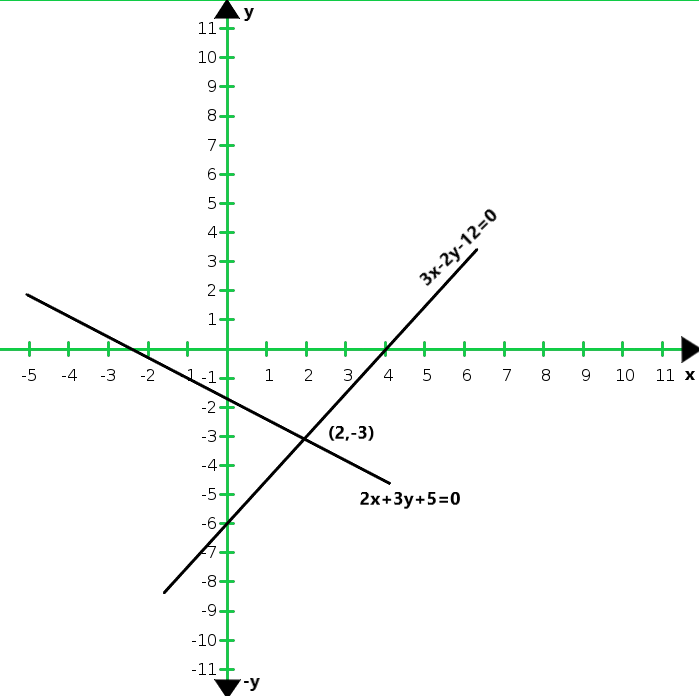From the graph we conclude that the two lines intersect at (2, 3).

Hence x = 2 and y = 3 are required points.

### 4x + 6y = 12

Solution:

Given that, 2x + 3y = 6 and 4x + 6y = 12

Now,  2x + 3y = 5

x = (6-3y)/2

When y = 0, we get x = 3

When y = 2, we get x = 0

So, the following table giving points on the line 2x + 3y = 6

Now,   4x + 6y = 12

x = (12 – 6y)/4

When y = 0, we get x = 3

When y = 2, we get x = 0

So, the following table giving points on the line 4x + 6y = 12

So, the graph of the equation 2x + 3y = 6 and 4x + 6y = 12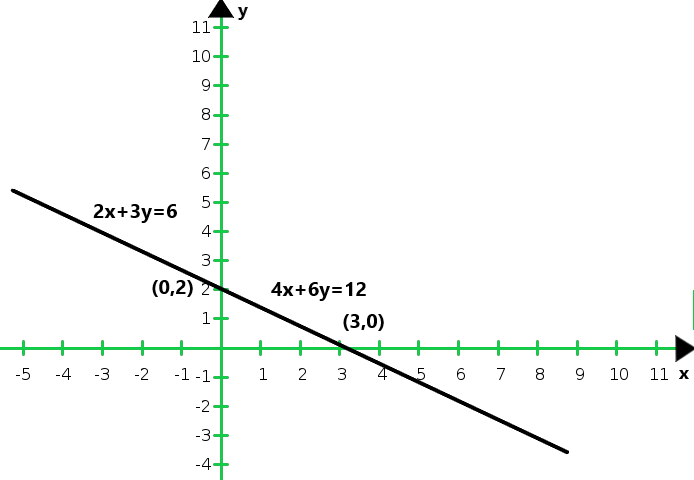From the graph we conclude that the two equations are coincident.

Hence, the system of equations has infinitely many solutions.

### 3x – 6y = 15

Solution:

Given that, x – 2y = 5 and 3x – 6y = 15

Now, x – 2y = 5

x = 2y + 5

When y = -1, we get x = 3

When y = 0, we get x = 5

So, the following table giving points on the line x – 2y = 5

Now, 3x – 6y = 15

x = (15 + 6y)/3

When y = -2, we get x = 1

When y = -3, we get x = -1

So, the following table giving points on the line 3x – 6y = 15

So, the graph of the equations x – 2y = 5 and 3x – 6y = 15: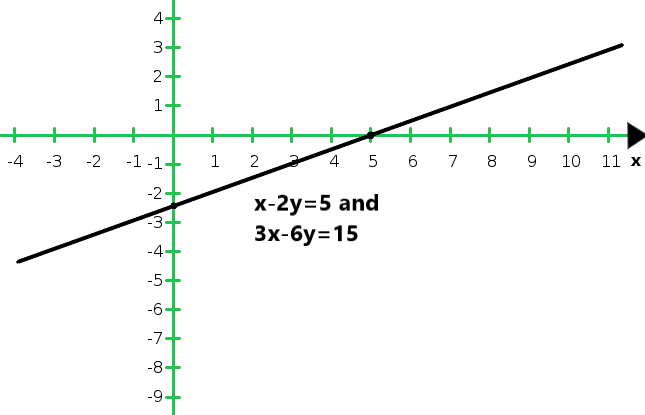From the graph we conclude that the two equations are coincident.

Hence, the system of equations has infinitely many solutions.

### 6x + 2y = 16

Solution:

Given that, 3x + y = 8 and 6x + 2y = 16

Now, x – 2y = 5

= y = 8 – 3x

When x = 2, we get y = 2

When x = 3, we get y = -1

So, the following table giving points on the line 3x + y = 8

Now, 6x + 2y = 16

y = (16 – 6x)/2

When x = 1, we get y = 5

When x = 3, we get y = -1

So, the following table giving points on the line 6x + 2y = 16

So, the graph of the given equations 3x + y = 8 and 6x + 2y = 16: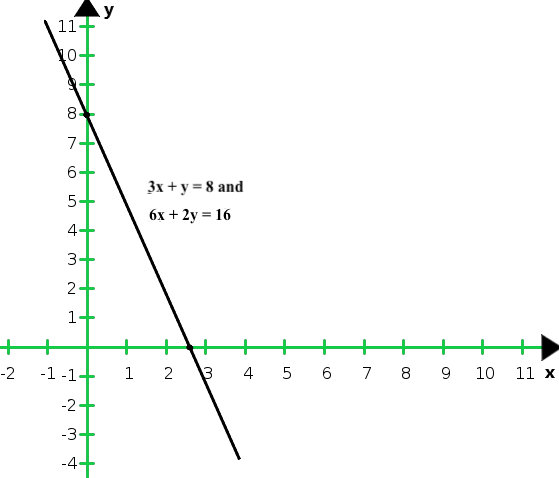From the graph we conclude that the graphs of the two equations are coincident

Hence, the system of equations has infinitely many solutions.

My Personal Notes arrow_drop_up
Related Tutorials